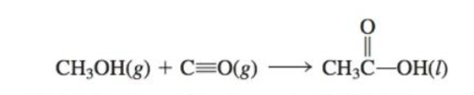# Compare your answer from Exercise 72 of Chapter 3 to the ∆H value calculated from standard enthalpies of formation in Appendix 4. Explain any discrepancies. ….. 72. Acetic acid is responsible for the sour taste of vinegar. It can be manufactured using the following reaction: Use tabulated values of bond engines(Table 3-3) to estimate ∆ E for this reaction### Chemistry: An Atoms First Approach

2nd Edition
Steven S. Zumdahl + 1 other
Publisher: Cengage Learning
ISBN: 9781305079243

#### Solutions

Chapter
Section### Chemistry: An Atoms First Approach

2nd Edition
Steven S. Zumdahl + 1 other
Publisher: Cengage Learning
ISBN: 9781305079243
Chapter 7, Problem 113AE
Textbook Problem
24 views

## Compare your answer from Exercise 72 of Chapter 3 to the ∆H value calculated from standard enthalpies of formation in Appendix 4. Explain any discrepancies.…..72. Acetic acid is responsible for the sour taste of vinegar. It can be manufactured using the following reaction:Use tabulated values of bond engines(Table 3-3) to estimate ∆E for this reaction

Interpretation Introduction

Interpretation: The comparative estimation of calculated enthalpy change and internal energy change of the given reaction should be explained.

Hess's Law:

Standard enthalpy of formation:

• The change in enthalpy that associate with the formation of one mole of a product from its pure elements, with all substances in its standard states is called as a standard enthalpy of formation.
• Formula:

ΔHfnpΔH°f(products)-nrΔH°f(reactants)......(1)

• Internal energy change of a reaction is given as,
• Internal energy change ΔE= Bond broken energy - Bond formation energy

ΔE=Dbroken-Dformed......(2)

• The internal energy change is equal to enthalpy change in the gas phase reactions.

### Explanation of Solution

Explanation

Record data from given:

CH3OH(g)+CO(g)CH3COOH(l)

C-Cbondenergy=347kJ/molC-Obondenergy=358kJ/mol CdoublebondO=745 kJ/molC triple bondO=1072kJ/mol

The given reactions and bond energies arerecorded as shown above.

To calculate the internal energy change of the given reactions.

ΔE=1072+358kJ-(347+745+358)=-20 kJ

• Thegiven bond energies are plugging in to above equation 2to get the internal energy change of the reactions.
• Internal energy change of reaction (a) = -20kJ .

To calculate the enthalpy change ΔΗ of given reactions

### Still sussing out bartleby?

Check out a sample textbook solution.

See a sample solution

#### The Solution to Your Study Problems

Bartleby provides explanations to thousands of textbook problems written by our experts, many with advanced degrees!

Get Started

Find more solutions based on key concepts
Why should units be carried along with numbers in a calculation?

General Chemistry - Standalone book (MindTap Course List)

Methylmercuryis a toxic industrial pollutant that is easily destroyed by cooking. T F

Nutrition: Concepts and Controversies - Standalone book (MindTap Course List)

What is the standard unit of volume in the SI?

An Introduction to Physical Science

In the following diagram, designate each daughter cell as diploid (2n) or haploid (n).

Human Heredity: Principles and Issues (MindTap Course List)

(a) At the time of this books printing, the U.S. national debt is about 16 trillion. If payments were made at t...

Physics for Scientists and Engineers, Technology Update (No access codes included)